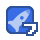# Exploring Mathematics -- Problem-Solving and Proof, Springer 2018.pdf21收藏

Introduction Have you ever faced a mathematical problem and had no idea how to approach it? Or perhaps you had an idea but got stuck halfway through? This book guides you in developing your creativity, as it takes you on a voyage of discovery into mathematics. Readers will not only learn strategies for solving problems and logical reasoning, but they will also learn about the importance of proofs and various proof techniques. Other topics covered include recursion, mathematical induction, graphs, counting, elementary number theory, and the pigeonhole, extremal and invariance principles. Designed to help students make the transition from secondary school to university level, this book provides readers with a refreshing look at mathematics and deep insights into universal principles that are valuable far beyond the scope of this book. Aimed especially at undergraduate and secondary school students as well as teachers, this book will appeal to anyone interested in mathematics. Only basic secondary school mathematics is required, including an understanding of numbers and elementary geometry, but no calculus. Including numerous exercises, with hints provided, this textbook is suitable for self-study and use alongside lecture courses. Keywords MSC (2010): 00-01, 00A07, 00A09, 97D50 problem solving mathematical proofs mathematical problem solving mathematical exploration problem solving strategies mathematics extremal principle invariance principle pigeonhole principle counting principles mathematical induction logic proofs elementary number theory Authors and affiliations Daniel Grieser1 1. Institut für MathematikCarl von Ossietzky Universität OldenburgOldenburgGermany

...展开详情

• 531
资源
• 18
粉丝
•等级
•签到新秀Exploring Mathematics -- Problem-Solving and Proof, Springer 2018.pdf 26积分/C币 立即下载
1/12726积分/C币 立即下载